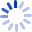Mathematical Statistics and Data Analysis

Mathematical Statistics and Data Analysis, Second Edition, introduces the important modern ideas of statistics into a mathematical statistics course, emphasizing data analysis, graphics, and computer-driven techniques. To show the integral role mathematical statistics plays in actual statistical practice and scientific investigations, the book examines real problems with real data to motivate your understanding of theory. In this edition, the author has integrated bootstrap material with more traditional inferential ...

Cash for Textbooks